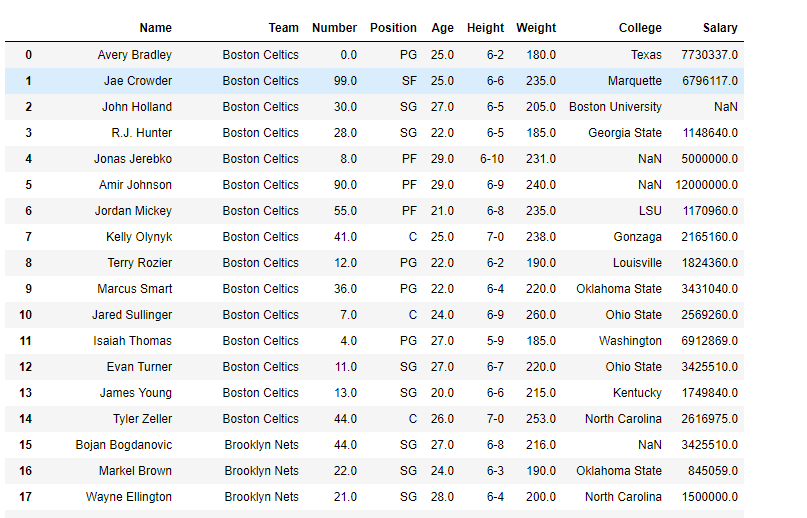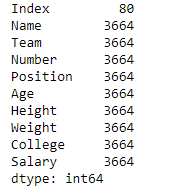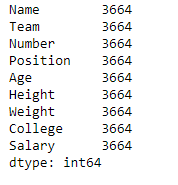# Python | Pandas dataframe.memory_usage()

• Last Updated : 22 Jun, 2021

Python is a great language for doing data analysis, primarily because of the fantastic ecosystem of data-centric python packages. Pandas is one of those packages and makes importing and analyzing data much easier.
Pandas dataframe.memory_usage() function return the memory usage of each column in bytes. The memory usage can optionally include the contribution of the index and elements of object dtype. This value is displayed in DataFrame.info by default.

Syntax: DataFrame.memory_usage(index=True, deep=False)
Parameters :
index : Specifies whether to include the memory usage of the DataFrame’s index in returned Series. If index=True the memory usage of the index the first item in the output.
deep : If True, introspect the data deeply by interrogating object dtypes for system-level memory consumption, and include it in the returned values.
Returns : A Series whose index is the original column names and whose values is the memory usage of each column in bytes

Example #1: Use memory_usage() function print the memory usage of each column in the dataframe along with the memory usage of the index.

## Python3

 `# importing pandas as pd` `import` `pandas as pd`   `# Creating the dataframe ` `df ``=` `pd.read_csv(``"nba.csv"``)`   `# Print the dataframe` `df`Let’s use the memory_usage() function to find the memory usage of each column.

## Python3

 `# Function to find memory use of each` `# column along with the index` `# even if we do not set index = True,` `# it will show the index usage as well by default.` `df.memory_usage(index ``=` `True``)`

Output :Example #2: Use memory_usage() function to find the memory use of each column but not of the index.

## Python3

 `# importing pandas as pd` `import` `pandas as pd`   `# Creating the dataframe ` `df ``=` `pd.read_csv(``"nba.csv"``)`   `# Function to find memory use of each` `# column but not of the index` `# we set index = False` `df.memory_usage(index ``=` `False``)`

Output :My Personal Notes arrow_drop_up
Recommended Articles
Page :